"
Last Updated: Thursday 30th December 2021

The aim of a programmer while creating a program is to ensure that the program stands the test for any random input. The program should correctly process the input and accurately calculate the output for any arbitrary data. A programmer must test the program for a wide range of information and amend the code accordingly to achieve this ambition.

But how do you choose a random number from the infinite possibilities available to you? Even if you have got a set of numbers, how do you change it into an efficient program? Are there any in-built functions to achieve this? Well, the answer is Yes!

Python offers a large number of modules and functions to use random data. This article will guide you to include these functions in your code and provide code snippets for your convenience. We’ll keep our range limited to {1,10} but remember, you can use these methods and programming syntax for any range you prefer. Let’s get started!

Python library used: Anaconda Distribution (Jupyter notebook)

## List Of Functions Available To Generate Random Numbers:

• random.randint() function
• random.randrange() function
• random.sample() function
• random.uniform() function
• numpy.random.randint() function
• numpy.random.uniform() function
• numpy.random.choice() function
• secrets.randbelow() function

### Using the ‘random.randint()’function:

random.randint() function is a part of the random module. The randint() function returns an integer value(of course, random!) between the starting and ending point entered in the function. This function is inclusive of both the endpoints entered.

We can write the code as follows: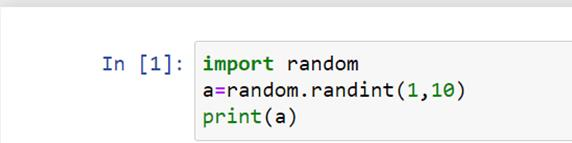The output of this program is as follows: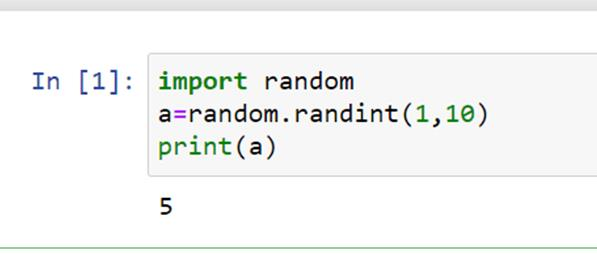The function returns the number 5 as a random output. Note that here we have generated only a single random number. You can also create a list of random numbers. The code for generating a list of random numbers is as shown below: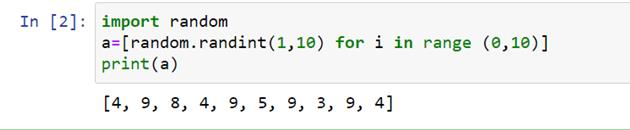The output is also shown in the code snippet given above. For loop can be used to generate a list.

### Using the ‘random.randrange()’function :

This function is similar to the randint() function. This function includes the step parameter and excludes the upper limit entered in the function. The step parameter is optional and is used to exclude a particular value in the given range. The default value of this parameter is 1.

The syntax for using the randrange() function is as follows: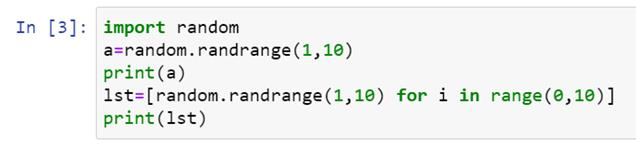The code given here prints the single random value as well as the list of random values.

The output is shown below: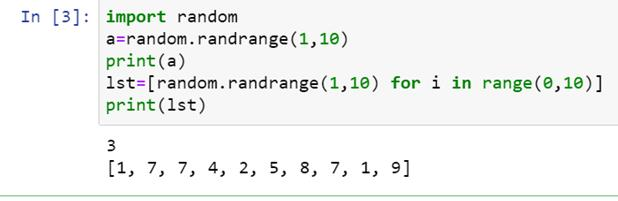As we can see here, the first line gives a single-digit output, whereas the following line gives a list of values.

### Using the ‘random.sample()’ function :

We use the sample() function to create a list that has the length of our choice. Also, it returns a list that has no repeated values.

We can write the code as follows: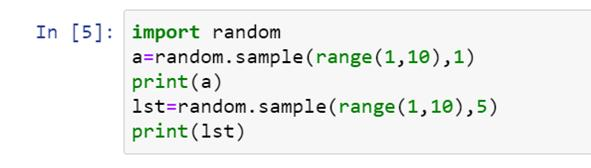Note that the syntax of this function uses the range() parameter to obtain the desired starting and ending values. Moreover, to generate a single-digit output, we have to enter ‘1’ as the list length beside the range() parameter.

The output we obtain is as follows: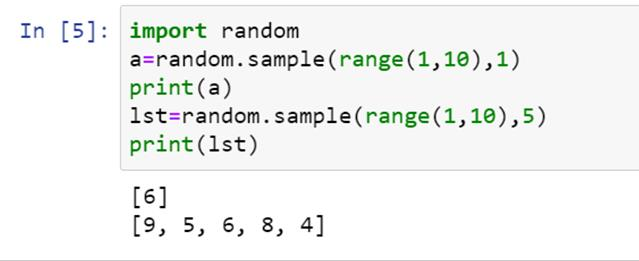### Using the ‘random.uniform()’ function :

We use the uniform() function to find floating-point numbers between the given range. This function is inclusive of both the endpoints of the given range.

The syntax of this code is the same as those mentioned for previous functions.The output of this code is a floating-point number.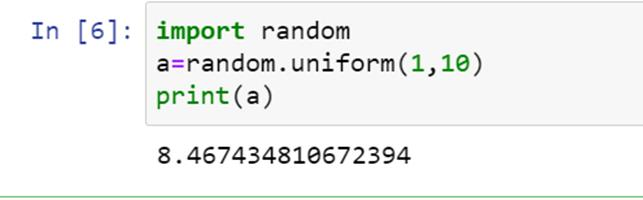To obtain the output as an integer, we can specially typecast the uniform() function.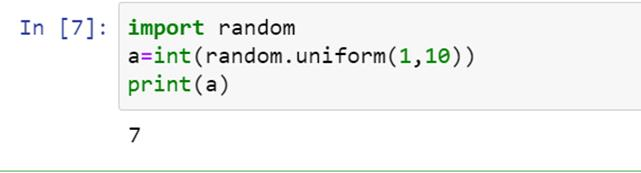Typecasting the function gives integer output.

### Using the‘numpy.random.randint()’function :

The numpy module also has the sub-module randomWe can use the numpy module when we want to generate a large number of numbers. This module stores the output in an array of the desired size.

The randint() method is used similarly as in the random module.

The syntax for this module is as follows: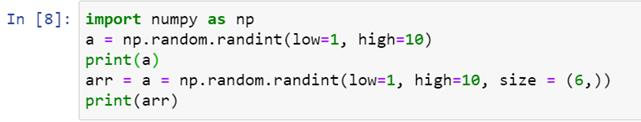In the output of this code, we will obtain an array of random numbers.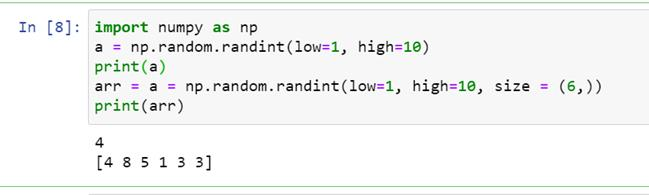As you can see, an array of size six is generated.

### Using the ‘numpy.random.uniform()’ function :

The numpy module also has the uniform() function to generate an array of floating-point numbers.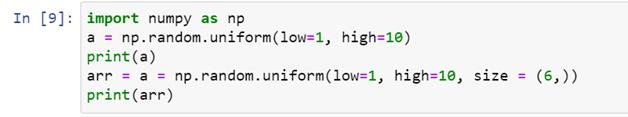Output: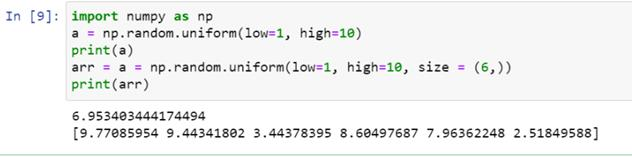### Using the ‘numpy.random.choice()’ function :

This function is used to obtain random numbers when we already have a list of numbers, and we have to choose a random number from that specific list. This function also stores the output in an array.

We can write the input of this function as follows: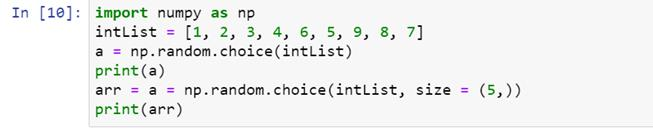Notice the arbitrary order of numbers in the user-entered list.

Let's see what the output of the given code is: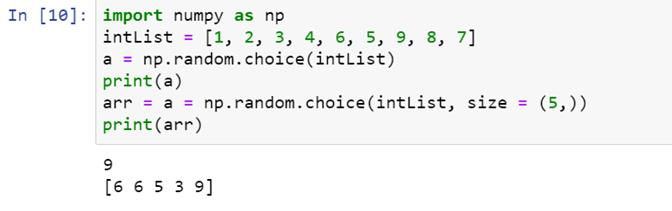### Using the ‘secrets.randbelow()’ function :

The secrets module is the most secure method to generate random numbers and finds excellent use in cryptography. One application of this module is to create strong, secure random passwords, tokens etc.

The randbelow()function is inclusive of both the limits entered in the function.

The syntax of this module is as follows:The output is shown below: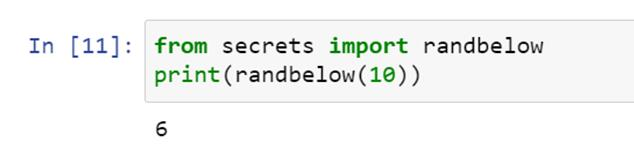## Conclusion

The use of random test cases is immense in programming. The built-in functions of several programming languages make it easier for us- the programmers to execute millions of arbitrary test cases and develop an efficient and accurate program. Python also offers a module for application in cryptography, as we have seen above. This module is beneficial if we want to create user-secure platforms. We hope this article helped you in learning the basics of random number generation.